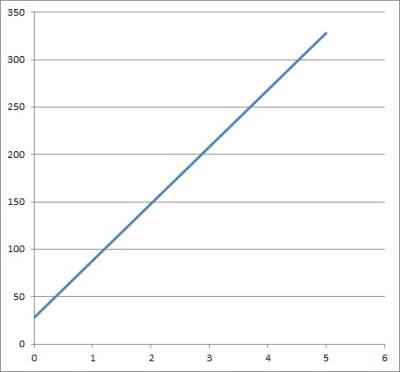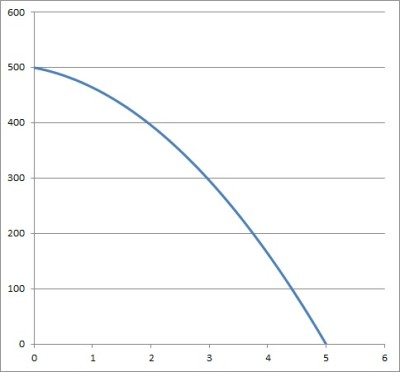# How To Solve An Initial Value Problem (5 Key Steps To Take)

Initial value problems come up often in calculus, physics, and other subjects.  You can solve some of them with straightforward antiderivatives, while others will require you to solve a challenging ordinary differential equation.

So, how do you solve an initial value problem?  First, write out the differential equation. Next, find the starting condition (initial value). Then, isolate the derivative in the equation. Now, take the antiderivative on both sides of the equation. Finally, use the starting condition (initial value) to find the constant in the antiderivative.

Of course, we can solve initial value problems in “layers”.  For example, in physics, we often start with acceleration (due to gravity) and find the height function for an object, given its starting height and speed.

In this article, we’ll talk about initial value problems and what they are.  We’ll also look at the steps you can take to solve them, along with some examples to show how it’s done in practice.

Let’s get started.

## What Is An Initial Value Problem?

An initial value problem (IVP) uses calculus, a differential equation, and a starting condition to find a function that models the problem.  For example, a common IVP in physics is to find an equation for the height of a falling object, given its starting height and velocity.

An initial value problem involves an ordinary differential equation (ODE).  An ordinary differential equation is made up of one or more functions and their derivatives.

Now that we know what an initial value problem is, let’s find out how to solve one.

## How To Solve An Initial Value Problem

There are five key steps you can take to help you solve an initial value problem.

• 1. Write out the equation – if the IVP is given as a word problem, you might have to translate into an equation.  Sometimes, the equation will be given.  You may need to introduce variables to write your equation.
• 2. Identify the starting condition – an IVP always has a starting condition (an initial value for the function).  Sometimes, the starting condition will be given.  Other times, you might have to use context to figure it out.
• 3. Isolate the derivative – before you can take an antiderivative (next step), you need to isolate the derivative function.  This is just like solving for any other variable in an equation.
• 4. Take the antiderivative – you will need to know the rules for taking antiderivatives of various types of functions.  This can get more complicated for advanced ordinary differential equations.
• 5. Use the starting condition – taking the antiderivative in the previous step will introduce an unknown constant.  We use the starting condition in our equation to find the value of the constant.

It will help to see these steps applied to a real problem, so let’s take a look at some now.

### Example 1: How To Solve An Initial Value Problem

Let’s say that you are somewhere due south of Boston.  You start driving south at a constant speed of 60 miles per hour.You can solve an initial value problem involving speed (unsigned velocity) to find position at a given time.

After 3.2 hours, you are 220 miles south of Boston.  How far from Boston were you after 1.5 hours?

To solve this problem, we’ll take the 5 steps listed above.

Step 1: write out the equation.

We are not given any variables, so we will need our own.  Let’s use S for the speed of the car, P for the position of the car, and t for the time (in hours).

The equation tells us the speed S of the vehicle at a given time t.  So, we have the equation:

• S(t) = 60

This suggests that the speed of the car is a constant 60 (miles per hour).

Remember that speed is the derivative of position, meaning S(t) = P’(t).  So, we can rewrite the equation as:

• P’(t) = 60

Step 2: identify the starting condition.

We are given that the car is 220 miles south of Boston after 3.2 hours.  This implies a position of P = 220 miles at a time t = 3.2 hours.

As an ordered pair, we can write this as (3.2, 220).

Step 3: isolate the derivative.

Since the derivative P’(t) is already isolated in the equation P’(t) = 60, we don’t need to do anything here.

Step 4: take the antiderivative.

This is not too difficult, since the antiderivative of a constant c is ct.  Taking the antiderivative on both sides of our equation gives us:

• ∫P’(t)dt = ∫60dt
• P(t) = 60t + K

where K is an unknown constant.

Step 5: use the starting condition.

Now, we use the starting condition to solve for the constant K.  Remember from Step 2 that our starting condition was P = 220 at t = 3.2.

Using these values in the equation we found in Step 4 gives us:

• P(t) = 60t + K
• P(3.2) = 60(3.2) + K
• 220 = 192 + K
• 220 – 192 = K
• 28 = K

So, the entire equation is P(t) = 60t + 28.  This tells us how many miles (south) the car is from Boston at t hours.The position of a car traveling at a constant speed is linear with respect to time. Here, we have the graph of P(t) = 60t + 28.

For example, at time t = 0, the car is P(0) = 60(0) + 28 = 28 miles south of Boston (that is, you started 28 miles south of Boston).

At time t = 1.5 hours, the car is P(1.5) = 60(1.5) + 28 = 90 + 28 = 118 miles south of Boston.

### Example 2: How To Solve An Initial Value Problem

Let’s try a classic initial value problem from physics.  This one has two “layers”, and two antiderivatives must be taken: the first to go from acceleration to velocity, and the second to go from velocity to position.

A ball is thrown downward from the top of a building.  The starting velocity is -20 feet per second (the negative denotes that it is falling, or moving towards Earth).  The starting height is 500 feet.Solving an initial value problem can tell you the height of a falling object at a time , given its starting height and velocity (starting velocity is zero if the ball is dropped from rest).

The acceleration due to gravity is -32 feet per second.  What is the height of the ball at 2.5 seconds?

Step 1: write out the equation.

We are not given any variables, so we will need our own.  Let’s use A for the acceleration of the ball, V for the velocity of the ball, P for the position (height) of the ball, and t for the time (in seconds).

The equation tells us the acceleration A of the ball at a given time t.  So, we have the equation:

• A(t) = -32

This suggests that the acceleration of the ball is a constant -32 (feet per second, per second).  This is the acceleration due to gravity.

Remember that acceleration is the derivative of velocity, meaning A(t) = V’(t).  So, we can rewrite the equation as:

• V’(t) = -32

Step 2: identify the starting condition.

We are given that the starting velocity of the ball is -20 feet per second.  This implies a velocity of V = -20 feet per second at a time t = 0 seconds.

As an ordered pair, we can write this as (0, -20).

Step 3: isolate the derivative.

Since the derivative V’(t) is already isolated in the equation V’(t) = -32, we don’t need to do anything here.

Step 4: take the antiderivative.

This is not too difficult, since the antiderivative of a constant c is ct.  Taking the antiderivative on both sides of our equation gives us:

• ∫V’(t)dt = ∫-32dt
• V(t) = -32t + K

where K is an unknown constant.

Step 5: use the starting condition.

Now, we use the starting condition to solve for the constant K.  Remember from Step 2 that our starting condition was V = -20 at t = 0.

Using these values in the equation we found in Step 4 gives us:

• P(t) = -32t + K
• P(0) = 60(0) + K
• -20 = 0 + K
• -20 = K

So, the entire equation is V(t) = -32t – 20.  This tells us the velocity of the ball at t seconds.

However, we’re not done yet.  We want to find the position function, so we have to go through the steps again (this time, to find position from velocity).

Step 1: write out the equation.

The equation we just found tells us the velocity V of the ball at a given time t.  So, we have the equation:

• V(t) = -32t – 20

Remember that velocity is the derivative of position, meaning V(t) = P’(t).  So, we can rewrite the equation as:

• P’(t) = -32t – 20

Step 2: identify the starting condition.

We are given that the starting position of the ball is a height of 500 feet above ground.  This implies a position of P = 500 feet at a time t = 0 seconds.

As an ordered pair, we can write this as (0, 500).

Step 3: isolate the derivative.

Since the derivative P’(t) is already isolated in the equation P’(t) = -32t – 20, we don’t need to do anything here.

Step 4: take the antiderivative.

This is not too difficult, since the antiderivative of a constant c is ct, and the antiderivative of a linear term bt is bt2/2.  Taking the antiderivative on both sides of our equation gives us:

• ∫P’(t)dt = ∫(-32t – 20)dt
• P(t) = -32t2/2 – 20t + K
• P(t) = -16t2 – 20t + K

where K is an unknown constant.

Step 5: use the starting condition.

Now, we use the starting condition to solve for the constant K.  Remember from Step 2 that our starting condition was P = 500 at t = 0.

Using these values in the equation we found in Step 4 gives us:

• P(t) = -16t2 – 20t + K
• P(0) = -16(0)2 – 20(0) + K
• 500 = 0 – 0 + K
• 500 = K

So, the entire equation is P(t) = -16t2 – 20t + 500.  This tells us the position of the ball at t seconds.The position function of a falling object is quadratic with respect to time. The graph is a parabola, and it highest at the starting point (and zero when it hits the ground). This is the graph of the function P(t) = -16t2 – 20t + 500.

The height of the ball at t = 2.5 seconds is given by:

• P(t) = -16t2 – 20t + 500
• P(2.5) = -16(2.5)2 – 20(2.5) + 500
• P(2.5) = -16(6.25) – 50 + 500
• P(2.5) = -100 + 450
• P(2.5) = 350

The ball is at a height of 350 feet above ground at 2.5 seconds.

## Conclusion

Now you know what an initial value problem is and how to solve one.  You also know the steps to take so that you have the right tools to solve the problem.

Velocity is used in other applications besides initial value problems – you can learn about what velocity is used for in this article.